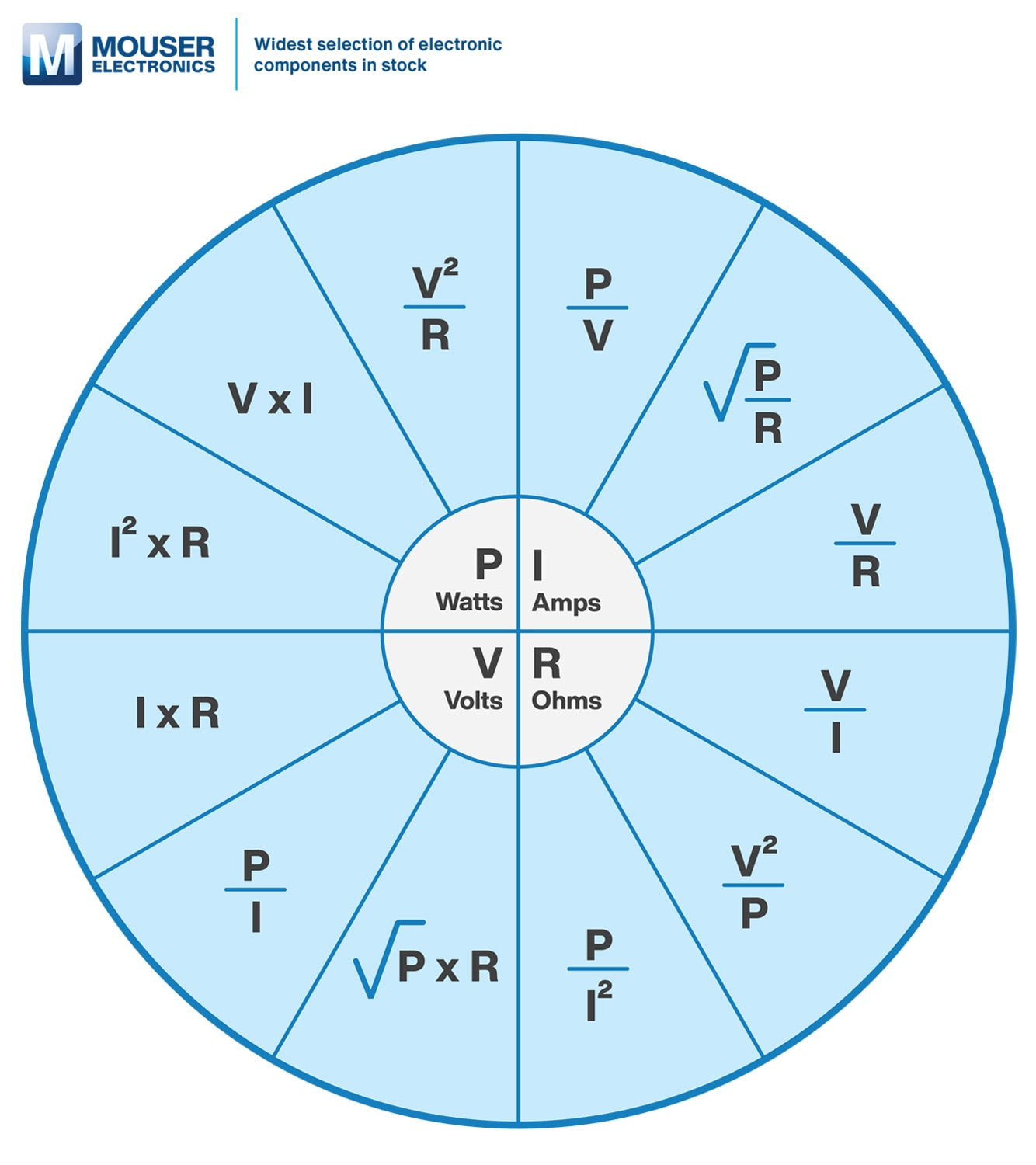Enter the 2 known values to solve for the remaining variables. For best results, reset the calculator after each use to solve different combinations of variables.

(V)

(A)

(Ω)

### Power

(W)
Ohm's Law Conversion Formula =
V = I x R

V

I R
I = V / R

V

I R
R = V / I

V

I R